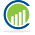## Saturday, 23 August 2014

### Calculating Mean in Hadoop MapReduce

#### Takes each input line and calculate the sum and stores the no of lines it sumed.Then sum get stored in a hash map with key as column Id.cleanup emits the sum and total line count inorder to take the overall mean.As we know each map only gets a block of input data.So while summing up we need to know how many elements summed up.

```//Calculating sum
if (sumVal.isEmpty()) {
//if sumval is empty add elements to sumval
sumVal.putAll(mapLine);
} else {
//calculating sum
double sum = 0;
for (Integer colId : mapLine.keySet()) {
double val1 = mapLine.get(colId);
double val2 = sumVal.get(colId);
/*
* calculating sum
*/
sum = val1 + val2;
sumVal.put(colId, sum);
}
}```

#### Reducer calculates 2 sums. Sums the values for each key and  Sums total no.of linecount

```for (TwovalueWritable value : values) {
//Taking sum of values and total number of lines
sum += value.getSum();
total += value.getTotalCnt();
}
//sum contains total sum of all elements in each column
//total contains total no of elements in each column
mean = sum / total;
valEmit.set(mean);
context.write(key, valEmit);```

#### This approach helps in avoiding a large no of communication with reducer.Reducer needs only to sum up few values from mapper.Say we have only 3 mappers and 4 columns in input set.Reducer only want to wait for 4 values from each mapper(no.of columns also considered)

Complete code : GitHub Link

#### 1 comment:

1.For latest and updated Cloudera certification dumps in PDF format contact us at completeexamcollection@gmail.com.
Refer our blog for more details http://completeexamcollection.blogspot.in/2015/04/cloudera-hadoop-certification-dumps.html